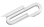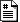# Jim'sTutorials

Spring 2018
##### site
• updated binary-tree.c to include a node typedef, a list typedef and some methods
• these methods include:
// function to print a linked list l starting at the first node
void printList(list l){
node n = l->first;    // n = first node
while(n != NULL){
printf(" %d ", n->data);
n = n->next;
};
};

// function to return a list struct with first and last node initiated as NULL and length 0
list createList(){
list new_list = (list)malloc(sizeof(struct node_list));
new_list->length = 0;
new_list->first = NULL;
new_list->last = NULL;
return new_list;
};

// function to push a given node n into the end of a given list l
void push(list l, node n){
if(l->length == 0){
l->first = n;
l->last = n;
l->length = 1;
} else {
l->last->next = n;
l->last = n;
l->length++;
}
}

// insert a new node n, with data - int data- after node prev
void insertAfter(node prev, int data){
node n = createNode(data);
n->next = prev->next;
prev->next = n;
}

• Implemented a basic binary tree that needs some refactoring
• created a function that recursively traverses the tree and prints the data in the nodes from left to right
• most of the code for this i found here: https://www.geeksforgeeks.org/tree-traversals-inorder-preorder-and-postorder/
• Tried to make a function that would take a linked list as an input and create a binary tree but didn't get that far and left the start of it in comments
https://cs.marlboro.college /cours /spring2018 /jims_tutorials /web_tech /c_apr10
## attachmentslast modified sizebinary-tree.c Tue Dec 05 2023 08:19 am 1.2Klinked-list.c Tue Dec 05 2023 08:19 am 2.3K